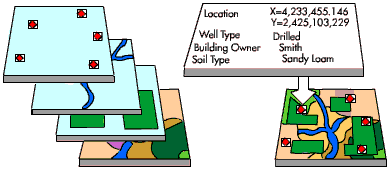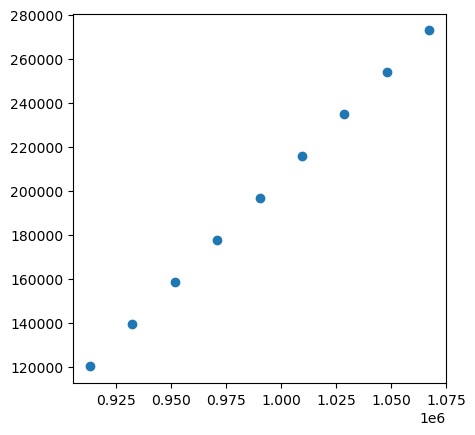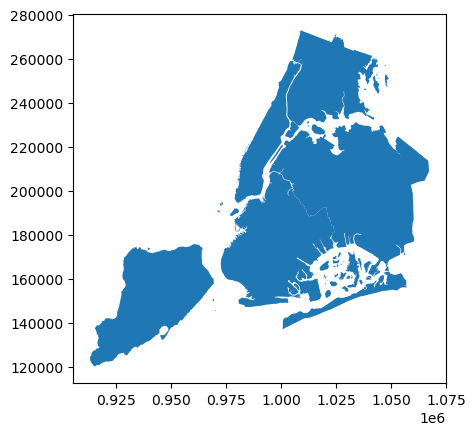Note

# Spatial Joins¶

A spatial join uses binary predicates such as intersects and crosses to combine two GeoDataFrames based on the spatial relationship between their geometries.

A common use case might be a spatial join between a point layer and a polygon layer where you want to retain the point geometries and grab the attributes of the intersecting polygons.## Types of spatial joins¶

We currently support the following methods of spatial joins. We refer to the left_df and right_df which are the correspond to the two dataframes passed in as args.

### Left outer join¶

In a LEFT OUTER JOIN (how='left'), we keep all rows from the left and duplicate them if necessary to represent multiple hits between the two dataframes. We retain attributes of the right if they intersect and lose right rows that don’t intersect. A left outer join implies that we are interested in retaining the geometries of the left.

This is equivalent to the PostGIS query:

SELECT pts.geom, pts.id as ptid, polys.id as polyid
FROM pts
LEFT OUTER JOIN polys
ON ST_Intersects(pts.geom, polys.geom);

geom                    | ptid | polyid
--------------------------------------------+------+--------
010100000048EABE3CB622D8BFA8FBF2D88AA0E9BF |    3 |     10
010100000048EABE3CB622D8BFA8FBF2D88AA0E9BF |    3 |     20
0101000000F0D88AA0E1A4EEBF7052F7E5B115E9BF |    2 |     20
(5 rows)


### Right outer join¶

In a RIGHT OUTER JOIN (how='right'), we keep all rows from the right and duplicate them if necessary to represent multiple hits between the two dataframes. We retain attributes of the left if they intersect and lose left rows that don’t intersect. A right outer join implies that we are interested in retaining the geometries of the right.

This is equivalent to the PostGIS query:

SELECT polys.geom, pts.id as ptid, polys.id as polyid
FROM pts
RIGHT OUTER JOIN polys
ON ST_Intersects(pts.geom, polys.geom);

geom    | ptid | polyid
----------+------+--------
01...9BF |    4 |     10
01...9BF |    3 |     10
02...7BF |    3 |     20
02...7BF |    2 |     20
00...5BF |      |     30
(5 rows)


### Inner join¶

In an INNER JOIN (how='inner'), we keep rows from the right and left only where their binary predicate is True. We duplicate them if necessary to represent multiple hits between the two dataframes. We retain attributes of the right and left only if they intersect and lose all rows that do not. An inner join implies that we are interested in retaining the geometries of the left.

This is equivalent to the PostGIS query:

SELECT pts.geom, pts.id as ptid, polys.id as polyid
FROM pts
INNER JOIN polys
ON ST_Intersects(pts.geom, polys.geom);

geom                    | ptid | polyid
--------------------------------------------+------+--------
010100000048EABE3CB622D8BFA8FBF2D88AA0E9BF |    3 |     10
010100000048EABE3CB622D8BFA8FBF2D88AA0E9BF |    3 |     20
0101000000F0D88AA0E1A4EEBF7052F7E5B115E9BF |    2 |     20
(4 rows)


## Spatial Joins between two GeoDataFrames¶

Let’s take a look at how we’d implement these using GeoPandas. First, load up the NYC test data into GeoDataFrames:

:

%matplotlib inline
from shapely.geometry import Point
from geopandas import datasets, GeoDataFrame, read_file

# NYC Boros
zippath = datasets.get_path('nybb')

# Generate some points
b = [int(x) for x in polydf.total_bounds]
N = 8
pointdf = GeoDataFrame([
{'geometry': Point(x, y), 'value1': x + y, 'value2': x - y}
for x, y in zip(range(b, b, int((b - b) / N)),
range(b, b, int((b - b) / N)))])

# Make sure they're using the same projection reference
pointdf.crs = polydf.crs

:

pointdf

:

geometry value1 value2
0 POINT (913175.000 120121.000) 1033296 793054
1 POINT (932450.000 139211.000) 1071661 793239
2 POINT (951725.000 158301.000) 1110026 793424
3 POINT (971000.000 177391.000) 1148391 793609
4 POINT (990275.000 196481.000) 1186756 793794
5 POINT (1009550.000 215571.000) 1225121 793979
6 POINT (1028825.000 234661.000) 1263486 794164
7 POINT (1048100.000 253751.000) 1301851 794349
8 POINT (1067375.000 272841.000) 1340216 794534
:

polydf

:

BoroCode BoroName Shape_Leng Shape_Area geometry
0 5 Staten Island 330470.010332 1.623820e+09 MULTIPOLYGON (((970217.022 145643.332, 970227....
1 4 Queens 896344.047763 3.045213e+09 MULTIPOLYGON (((1029606.077 156073.814, 102957...
2 3 Brooklyn 741080.523166 1.937479e+09 MULTIPOLYGON (((1021176.479 151374.797, 102100...
3 1 Manhattan 359299.096471 6.364715e+08 MULTIPOLYGON (((981219.056 188655.316, 980940....
4 2 Bronx 464392.991824 1.186925e+09 MULTIPOLYGON (((1012821.806 229228.265, 101278...
:

pointdf.plot()

:

<AxesSubplot:>:

polydf.plot()

:

<AxesSubplot:>## Joins¶

:

join_left_df = pointdf.sjoin(polydf, how="left")
join_left_df
# Note the NaNs where the point did not intersect a boro

:

geometry value1 value2 index_right BoroCode BoroName Shape_Leng Shape_Area
0 POINT (913175.000 120121.000) 1033296 793054 NaN NaN NaN NaN NaN
1 POINT (932450.000 139211.000) 1071661 793239 0.0 5.0 Staten Island 330470.010332 1.623820e+09
2 POINT (951725.000 158301.000) 1110026 793424 0.0 5.0 Staten Island 330470.010332 1.623820e+09
3 POINT (971000.000 177391.000) 1148391 793609 NaN NaN NaN NaN NaN
4 POINT (990275.000 196481.000) 1186756 793794 NaN NaN NaN NaN NaN
5 POINT (1009550.000 215571.000) 1225121 793979 1.0 4.0 Queens 896344.047763 3.045213e+09
6 POINT (1028825.000 234661.000) 1263486 794164 4.0 2.0 Bronx 464392.991824 1.186925e+09
7 POINT (1048100.000 253751.000) 1301851 794349 NaN NaN NaN NaN NaN
8 POINT (1067375.000 272841.000) 1340216 794534 NaN NaN NaN NaN NaN
:

join_right_df = pointdf.sjoin(polydf, how="right")
join_right_df
# Note Staten Island is repeated

:

index_left value1 value2 BoroCode BoroName Shape_Leng Shape_Area geometry
0 1.0 1071661.0 793239.0 5 Staten Island 330470.010332 1.623820e+09 MULTIPOLYGON (((970217.022 145643.332, 970227....
0 2.0 1110026.0 793424.0 5 Staten Island 330470.010332 1.623820e+09 MULTIPOLYGON (((970217.022 145643.332, 970227....
1 5.0 1225121.0 793979.0 4 Queens 896344.047763 3.045213e+09 MULTIPOLYGON (((1029606.077 156073.814, 102957...
2 NaN NaN NaN 3 Brooklyn 741080.523166 1.937479e+09 MULTIPOLYGON (((1021176.479 151374.797, 102100...
3 NaN NaN NaN 1 Manhattan 359299.096471 6.364715e+08 MULTIPOLYGON (((981219.056 188655.316, 980940....
4 6.0 1263486.0 794164.0 2 Bronx 464392.991824 1.186925e+09 MULTIPOLYGON (((1012821.806 229228.265, 101278...
:

join_inner_df = pointdf.sjoin(polydf, how="inner")
join_inner_df
# Note the lack of NaNs; dropped anything that didn't intersect

:

geometry value1 value2 index_right BoroCode BoroName Shape_Leng Shape_Area
1 POINT (932450.000 139211.000) 1071661 793239 0 5 Staten Island 330470.010332 1.623820e+09
2 POINT (951725.000 158301.000) 1110026 793424 0 5 Staten Island 330470.010332 1.623820e+09
5 POINT (1009550.000 215571.000) 1225121 793979 1 4 Queens 896344.047763 3.045213e+09
6 POINT (1028825.000 234661.000) 1263486 794164 4 2 Bronx 464392.991824 1.186925e+09

We’re not limited to using the intersection binary predicate. Any of the Shapely geometry methods that return a Boolean can be used by specifying the op kwarg.

:

pointdf.sjoin(polydf, how="left", op="within")

/home/docs/checkouts/readthedocs.org/user_builds/geopandas/conda/stable/lib/python3.9/site-packages/IPython/core/interactiveshell.py:3441: FutureWarning: The op parameter is deprecated and will be removed in a future release. Please use the predicate parameter instead.
exec(code_obj, self.user_global_ns, self.user_ns)

:

geometry value1 value2 index_right BoroCode BoroName Shape_Leng Shape_Area
0 POINT (913175.000 120121.000) 1033296 793054 NaN NaN NaN NaN NaN
1 POINT (932450.000 139211.000) 1071661 793239 0.0 5.0 Staten Island 330470.010332 1.623820e+09
2 POINT (951725.000 158301.000) 1110026 793424 0.0 5.0 Staten Island 330470.010332 1.623820e+09
3 POINT (971000.000 177391.000) 1148391 793609 NaN NaN NaN NaN NaN
4 POINT (990275.000 196481.000) 1186756 793794 NaN NaN NaN NaN NaN
5 POINT (1009550.000 215571.000) 1225121 793979 1.0 4.0 Queens 896344.047763 3.045213e+09
6 POINT (1028825.000 234661.000) 1263486 794164 4.0 2.0 Bronx 464392.991824 1.186925e+09
7 POINT (1048100.000 253751.000) 1301851 794349 NaN NaN NaN NaN NaN
8 POINT (1067375.000 272841.000) 1340216 794534 NaN NaN NaN NaN NaN